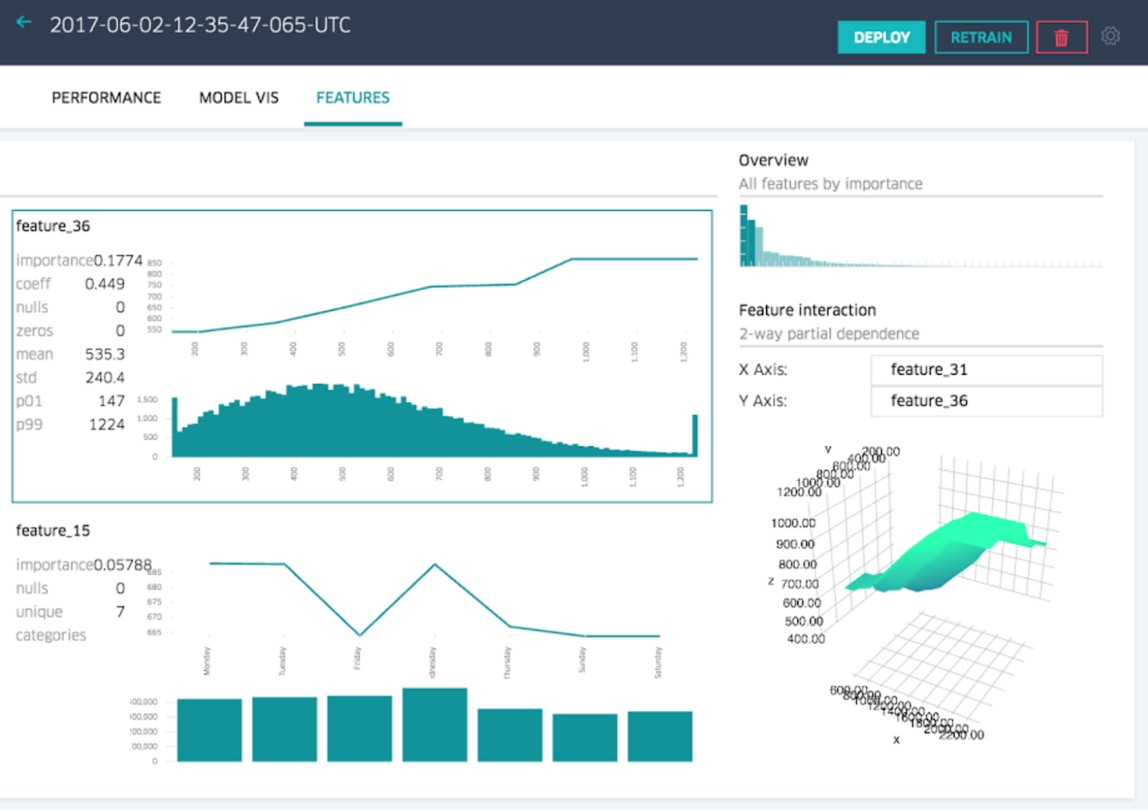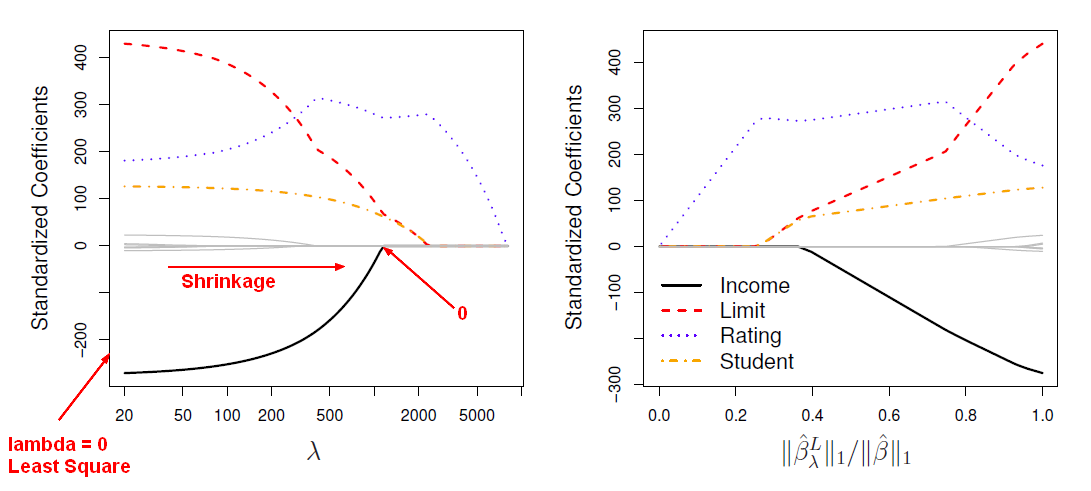# Statistics - Best Subset Selection Regression

The most direct approach in order to generate a set of model for the feature selection approach is called all subsets or best subsets regression. We compute the least squares t for all possible subsets in order to choose them.

## Procedure

The natural way to do it is to consider every possible subset of p predictors and to choose the best model out of every single model the just contains some of the predictors.

1. Calculate the null model noted as $M_0$ . 0 for 0 parameters.
2. Select the best model with one predictor called $M_1$ . In order to get $M_1$ , we fit all p models that contain exactly one predictor $(^p_1)$ .
3. Fit all model $(^p_2)$ and select the best model with exactly 2 predictor called $M_2$
4. ….
5. Fit all model $(^p_k)$ and select the best model with p predictors $M_p$ .

This procedure produces a path of models.

The best model is defined by type of model:

This notation $(^p_k)$ is pronounced “choose” and means p choose k. It is the number of possible models that I can get that contain exactly k predictors out of p predictors total. $$\begin{array}{rrc} \left(^p_k \right) & = & \frac{p!}{k!(p-k)!} \end{array}$$

## Best subset Issues

Best subset selection suffers from:

• Computational Performance

If I have access to p predictors, and I want to consider every possible sub model, I'm actually looking at 2 to the p subsets. That is an absolutely huge number. When p is large, we're really not going to be able to do best subset selection. 40 predictors isn't large. Actually, there's easily tens or hundreds of thousands of predictors. So, Best subset selection just does not scale for many of the types of problems that people are interested in today. The limit for most R packages or function that do subset selection is about 30 or 40.

• Statistical problems: Generalization, Overfitting

If I have 40 predictors, and I'm considering a trillion models (A Gosh), I'm going to over fit the data because a I'm just looking at so many models, I'm going to choose a model that looks really, really great on the training data but it's not going to look great on an independent test. It's a reasonable thing to do with a small number of variables, but it gets really hard when the number of variables gets large. Each predictor can either be included or not included in the model. That means that for each of the p variables there are two options. Number of Subset: $2^p$ . 2 to the p grows exponentially with the number of variables.

For these two reasons– computational and statistical– best subset selection isn't really great unless p is extremely small. Best Subset Selection is rarely used in practice for say p=10 or larger.

Discover MoreData Mining - (Attribute|Feature) (Selection|Importance)

Feature selection is the second class of dimension reduction methods. They are used to reduce the number of predictors used by a model by selecting the best d predictors among the original p predictors....R - Feature selection - Model Generation (Best Subset and Stepwise)

This article talks the first step of feature selection in R that is the models generation. Once the models are generated, you can select the best model with one of this approach: Best...R - Shrinkage Method (Ridge Regression and Lasso)

Unlike subset and forward stepwise regression, which controls the complexity of a model by restricting the number of variables, ridge regression keeps all the variables in and shrinks the coefficients...Statistical Learning - Lasso

Lasso is a shrinkage method. Ridge regression doesn't actually select variables by settings the parameters to zero. Lasso is a more recent technique for shrinking coefficients in regression that overcomes...Statistics - Forward and Backward Stepwise (Selection|Regression)

In statistics, stepwise regression includes regression models in which the choice of predictive variables is carried out by an automatic procedure. Stepwise methods have the same ideas as best subset...